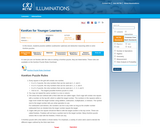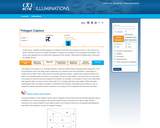Updating search results...

# 11 Results

View
Selected filters:
• NCTM IlluminationsRating
0.0 stars

This resource is an example of modeling the line of best fit by plotting the number of rubber bands that form a bungee cord and how far a Barbie doll can bungee jump with the length of cord. Students calculate the line of best fit by hand and using a graphing calculator. They interpret key parts of the line such as slope and y-intercept.

Subject:
Mathematics
Material Type:
Interactive
Lesson
Lesson Plan
Provider:
NCTM Illuminations
10/09/2017Only Sharing Permitted
CC BY-NC-ND
Rating
0.0 stars

Students will simulate a jumping frog contest and collect data for frog distances jumped.  Students will measure three consecutive jumps along with the total distance jumped in centimeters.  Students will determine the range of classroom data jumps and find the mean and the median of jump data.

Subject:
Mathematics
Material Type:
Lesson Plan
Simulation
Provider:
NCTM Illuminations
04/20/2016Restricted Use
Rating
0.0 stars

Explore the different conic sections and their graphs. Use the Cone View to manipulate the cone and the plane creating the cross section, and then observe how the Graph View changes.

Subject:
Mathematics
Material Type:
Activity/Lab
Diagram/Illustration
Formative Assessment
Interactive
Self Assessment
Simulation
Provider:
NCTM Illuminations
11/03/2016Restricted Use
Rating
0.0 stars

Create equivalent fractions by dividing and shading squares or circles, and match each fraction to its location on the number line.

Subject:
Mathematics
Material Type:
Activity/Lab
Diagram/Illustration
Interactive
Self Assessment
Simulation
Provider:
NCTM Illuminations
02/14/2017Restricted Use
Rating
0.0 stars

Students extend their knowledge of proportions to solving problems dealing with similarity. They measure the heights and shadows of familiar objects and use indirect measurement to find the heights of things that are much bigger in size, such as a flagpole, a school building, or a tree.

Subject:
Mathematics
Material Type:
Lesson Plan
Provider:
NCTM Illuminations
11/17/2015Conditional Remix & Share Permitted
CC BY-NC
Rating
0.0 stars

In this lesson, students practice addition (subtraction optional) and deductive reasoning skills to solve KenKen puzzles, which is a break off of the Sudoku puzzle.  All of the directions of what a KenKen puzzle is and how to use them are included in the resource in detail.  The students investigate as a group what they think is happening in a completed puzzle.  In this way they are using the Math Practice Standards MP1 and MP7.  Once they understand the rules, they will work on solving problems using addition and possibly subtraction.

Subject:
Mathematics
Material Type:
Formative Assessment
Game
Lesson Plan
Provider:
NCTM Illuminations
12/15/2016Restricted Use
Rating
0.0 stars

Students will explore estimation of length.
K student will use the non standard unit (ladybugs) to measure the length of something longer than the given unit (e.g., their workspace).
There are suggestions to vary the lesson for each grade level (K-2).
There are other related resources linked within this lesson.

Subject:
Mathematics
Material Type:
Activity/Lab
Lesson Plan
Simulation
Provider:
NCTM Illuminations- Resources for Teaching Math
06/16/2015Restricted Use
Rating
0.0 stars

This applet allows the user to investigate the mean, median, and box-and-whisker plot for a set of data that they create. The data set may contain up to 15 integers, each with a value from 0 to 100.

Subject:
Mathematics
Material Type:
Activity/Lab
Diagram/Illustration
Formative Assessment
Interactive
Simulation
Provider:
NCTM Illuminations
11/30/2016Restricted Use
Rating
0.0 stars

In this two-player e-example, students take timed turns racing to the end of each fraction line by moving one or more of their markers to sum to a given fraction value.

Subject:
Mathematics
Material Type:
Activity/Lab
Diagram/Illustration
Interactive
Self Assessment
Provider:
NCTM Illuminations
02/14/2017Restricted Use
Rating
0.0 stars

In the game Polygon Capture, students select polygons based on specific attributes as associated with angles of the polygon and sides of the polygons.  During the activity, students will be presented with situations they may not always encounter in geomoetry.  For instance, instead of being asked to select "all polygons with right angles" and "all polygons with 4 equal sides" they may be asked to select "all polygons with at least one right angle" and with "no parallel sides."

Subject:
Mathematics
Material Type:
Formative Assessment
Game
Provider:
NCTM Illuminations
10/06/2015Restricted Use
Rating
0.0 stars

Students are encouraged to build conceptual reasoning for multiplying decimals by manipulating an area model.

Subject:
Mathematics
Material Type:
Activity/Lab
Diagram/Illustration
Interactive